Name: Krista Bischoff Who is asking: Student Question: One corner of a page of width a is folded over and just reaches the opposite side. Express L, the length of the crease, in terms of x and a. I can't get the picture to copy to this form so I guess I will have to try and describe the picture the best that I can. The top right hand corner is folded to the left side, almost half way down. The width of the paper is a ( the width of the bottom part which is not folded.) The creased side is L and the part shorter part of the folded area is x (the part that would have been the top right of the original piece.) I hope that this is good enough for you to understand. Hi Krista, In the diagram below the point P is folded to the point S leaving the "crease" QT of length L. PS is the line segment joing P and S.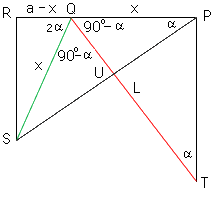Triangles PQU and QSU are congruent and thus angle QUP = angle SUQ. Since their sum is 180o they are each 90o angles. If angle QPU = angle USQ = a then angle PQU =angle UQS = 90o - a. Therefore angle SQR = 2a. Using triangle SQR we see that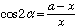Using the fact that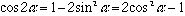we see that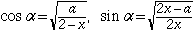Also from triangle PQT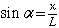Thus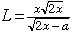Cheers, Chris Go to Math Central